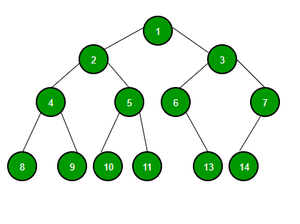# Test Case Generation | Set 6 (Random Unweighted Binary Tree)

• Last Updated : 15 Jun, 2021

Generating Random Unweighted Binary Tree:

• Since this is a tree, the test data generation plan is such that no cycle gets formed.
• The number of edges is one less than the number of vertices.
• For each RUN, first print the count of nodes say, N and the next N – 1 lines are of the form (a, b) where a is the parent of b.
• Each node contains at most 2 children.Approach: The problem can be solved using Queue. The idea is to traverse the tree using BFS. Follow the steps below to solve the problem:

• Initialize a map, say mp to check if a node is already included in the tree or not.
• Initialize a queue to store the nodes at each level of the tree.
• Consider 1 as root node and insert it into the queue.
• Iterate over the queue while the total count of nodes in the tree not equal to N. In every iteration insert distinct nodes of each level of the tree into the queue using rand() function and the map, also insert the node and the parent of the node into an array
• Finally, print the value of N and the array.

## CPP

 `// C++ Program to generate test cases for``// an unweighted tree`` ` `#include ``using` `namespace` `std;`` ` ` ` `// Function to generate the binary tree using BFS``vector > generateBinaryTree(``int` `n)``{``     ` `     ` `    ``// Stores number of children``    ``// a node can have``    ``vector<``int``> options = { 0, 1, 2 };``     ` `     ` `    ``// Check if a node is already``    ``// included in the tree or not``    ``map<``int``, ``int``> mp;`` ` ` ` `    ``// Stores node of tree at ``    ``// each level of the tree``    ``queue<``int``> q;`` ` ` ` `    ``// Insert root node``    ``q.push(1);`` ` ` ` `    ``// Stores the generated tree``    ``vector > v;`` ` ` ` `    ``// Store count of nodes``    ``// already included``    ``int` `count = 1;`` ` ` ` `    ``// Marking the inclusion``    ``// of node 1``    ``mp = 1;`` ` ` ` `    ``// Traverse tree using BFS``    ``while` `(!q.empty() or count < n) {``         ` `         ` `        ``// Stores from element``        ``// of queue``        ``int` `front;``         ` `        ``if``(!q.empty()) {``             ` `             ` `            ``// Update front``            ``front = q.front();``             ` `             ` `            ``// Pop front element ``            ``// of queue``            ``q.pop();``        ``}``         ` `         ` `         ` ` ` ` ` `        ``// Find count of child nodes``        ``// of current node``        ``int` `numberOfChilds``          ``= options[``rand``() % (options.size())];`` ` ` ` `        ``// If all the nodes are ``        ``// already included``        ``if` `(count >= n)``            ``continue``;``             ` ` ` `        ``// Connect child node to``        ``// the parent node``        ``while` `(numberOfChilds--) {``             ` `             ` `            ``// Stores value in node which``            ``// is not already included``            ``int` `child = ``rand``() % n + 1;`` ` ` ` `            ``// Find the child until found a node``            ``// that is not yet included``            ``while` `(mp[child]) {``                ``child++;``                ``if` `(child > n) {``                    ``child = 1;``                ``}``            ``}`` ` `             ` `            ``// Update count``            ``count++;`` ` ` ` `            ``// Mark the included node``            ``mp[child] = 1;`` ` ` ` `            ``// Insert it to the generated tree``            ``// as {parent, child}``            ``v.push_back({ front, child });`` ` ` ` `            ``// Push the child into the queue``            ``q.push(child);`` ` ` ` `            ``// If all the nodes are included``            ``// break``            ``if` `(count == n)``                ``break``;``        ``}``    ``}`` ` ` ` `    ``// Shuffle the v vector randomly``    ``random_shuffle(v.begin(), v.end());`` ` `    ``return` `v;``}`` ` ` ` `// Function to print the generated tree``void` `printTree(``int` `n, vector > v)``{``    ``int` `s = v.size();`` ` ` ` `    ``// Number of nodes``    ``cout << n << ``"\n"``;`` ` ` ` `    ``// Print n-1 edges as {parent, child}``    ``for` `(``int` `i = 0; i < v.size(); i++) {``        ``cout << v[i].first << ``" "` `<< v[i].second << ``"\n"``;``    ``}``}`` ` ` ` `// Driver Code``int` `main()``{``     ` `     ` `    ``// Random seeding``    ``srand``(``time``(NULL));`` ` ` ` ` ` `    ``// Number of node between 3 to 8``    ``// this range can be easily changed``    ``int` `n = ``rand``() % 6 + 3;`` ` ` ` `    ``// Function Call``    ``vector > v ``              ``= generateBinaryTree(n);`` ` `    ``// Print the generated tree``    ``printTree(n, v);``}`

Output:

```5
5 4
1 2
1 3
3 5
```

My Personal Notes arrow_drop_up